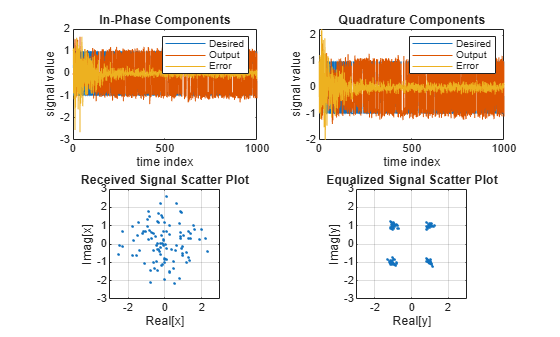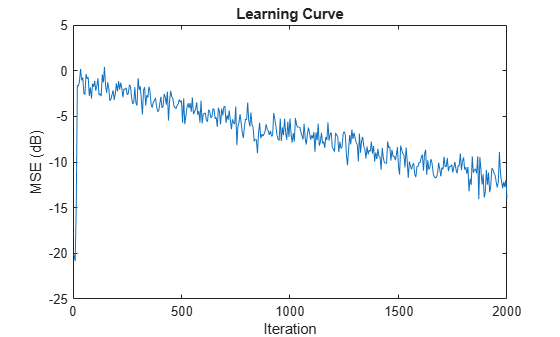# dsp.AffineProjectionFilter

Compute output, error and coefficients using affine projection (AP) Algorithm

## Description

The `dsp.AffineProjectionFilter` System object™ filters each channel of the input using AP filter implementations.

To filter each channel of the input:

1. Create the `dsp.AffineProjectionFilter` object and set its properties.

2. Call the object with arguments, as if it were a function.

## Creation

### Syntax

``apf = dsp.AffineProjectionFilter``
``apf = dsp.AffineProjectionFilter(len)``
``apf = dsp.AffineProjectionFilter(Name,Value)``

### Description

````apf = dsp.AffineProjectionFilter` returns an adaptive FIR filter System object, `apf`. This System object computes the filtered output and the filter error for a given input and desired signal using the affine projection (AP) algorithm. ```

example

````apf = dsp.AffineProjectionFilter(len)` returns an affine projection filter object with the `Length` property set to `len`.```

example

````apf = dsp.AffineProjectionFilter(Name,Value)` returns an affine projection filter object with each specified property set to the specified value. Enclose each property name in single quotes. Unspecified properties have default values.```

## Properties

expand all

Unless otherwise indicated, properties are nontunable, which means you cannot change their values after calling the object. Objects lock when you call them, and the `release` function unlocks them.

If a property is tunable, you can change its value at any time.

Specify the method used to calculate filter coefficients as ```Direct Matrix Inversion```, `Recursive Matrix Update`, ```Block Direct Matrix Inversion```. This property is nontunable.

Specify the length of the FIR filter coefficients vector as a scalar positive integer value. This property is nontunable.

Data Types: `single` | `double` | `int8` | `int16` | `int32` | `int64` | `uint8` | `uint16` | `uint32` | `uint64`

Specify the projection order of the affine projection algorithm as a scalar positive integer value greater than or equal to 2. This property defines the size of the input signal covariance matrix. This property is nontunable.

Data Types: `double`

Specify the affine projection step size factor as a scalar nonnegative numeric value between 0 and 1, both inclusive. Setting the step size equal to one provides the fastest convergence during adaptation.

Tunable: Yes

Data Types: `single` | `double` | `int8` | `int16` | `int32` | `int64` | `uint8` | `uint16` | `uint32` | `uint64` | `logical`

Specify the initial values of the FIR adaptive filter coefficients as a scalar or a vector of length equal to the `Length` property value.

Tunable: Yes

Data Types: `single` | `double` | `int8` | `int16` | `int32` | `int64` | `uint8` | `uint16` | `uint32` | `uint64`

Specify the initial values for the offset input covariance matrix. This property must be either a scalar positive numeric value or a positive-definite square matrix with each dimension equal to the `ProjectionOrder` property value. If it is a scalar value, the `OffsetCovariance` property is initialized to a diagonal matrix with the diagonal elements equal to that scalar value. If it is a square matrix, the` OffsetCovariance` property is initialized to the value of that square matrix.

Tunable: Yes

#### Dependencies

This property is applicable only if the `Method` property is set to `Direct Matrix Inversion` or ```Block Direct Matrix Inversion```.

Data Types: `single` | `double` | `int8` | `int16` | `int32` | `int64` | `uint8` | `uint16` | `uint32` | `uint64`

Specify the initial values for the offset input covariance matrix inverse. This property must be either a scalar positive numeric value or a positive-definite square matrix with each dimension equal to the `ProjectionOrder` property value. If it is a scalar value, the `InverseOffsetCovariance` property is initialized to a diagonal matrix with each diagonal element equal to that scalar value. If it is a square matrix, the `InverseOffsetCovariance` property is initialized to the values of that square matrix.

Tunable: Yes

#### Dependencies

This property is applicable only if the `Method` property is set to `Recursive Matrix Update`.

Data Types: `single` | `double` | `int8` | `int16` | `int32` | `int64` | `uint8` | `uint16` | `uint32` | `uint64`

Specify the initial values of the correlation coefficients of the FIR filter as a scalar or a vector of length equal to `ProjectionOrder``1`.

Tunable: Yes

#### Dependencies

This property is applicable only if the `Method` property is set to `Recursive Matrix Update`.

Data Types: `single` | `double` | `int8` | `int16` | `int32` | `int64` | `uint8` | `uint16` | `uint32` | `uint64`

Specify whether the filter coefficient values should be locked. When you set this property to `true`, the filter coefficients are not updated and their values remain the same. The default value is `false` (filter coefficients are continuously updated).

Tunable: Yes

## Usage

### Syntax

``[y,err] = apf(x,d)``

### Description

example

``` `[y,err] = apf(x,d)` filters the input `x`, using `d` as the desired signal, and returns the filtered output in `y` and the filter error in `err`. The System object estimates the filter weights needed to minimize the error between the output signal and the desired signal. You can access these coefficients by accessing the `Coefficients` property of the object. This can be done only after calling the object. For example, to access the optimized coefficients of the `apf` filter, call `apf.Coefficients` after you pass the input and desired signal to the object.```

### Input Arguments

expand all

The signal to be filtered by the affine projection filter. The input, `x`, and the desired signal, `d`, must have the same size and data type.

The input can be a variable-size signal. You can change the number of elements in the column vector even when the object is locked. The System object locks when you call the object to run its algorithm.

Data Types: `single` | `double`
Complex Number Support: Yes

The affine projection filter adapts its coefficients to minimize the error, `err`, and converge the input signal `x` to the desired signal `d` as closely as possible.

The input, `x`, and the desired signal, `d`, must have the same size and data type.

The desired signal can be a variable-size signal. You can change the number of elements in the column vector even when the object is locked. The System object locks when you call the object.

Data Types: `single` | `double`
Complex Number Support: Yes

### Output Arguments

expand all

Filtered output, returned as a scalar or a column vector. The object adapts its filter coefficients to converge the input signal `x` to match the desired signal `d`. The filter outputs the converged signal.

Data Types: `single` | `double`
Complex Number Support: Yes

Difference between the output signal `y` and the desired signal `d`, returned as a scalar or a column vector. The objective of the affine projection filter is to minimize this error. The object adapts its coefficients to converge towards optimal filter coefficients that produce an output signal that matches closely with the desired signal. To access the affine projection filter coefficients, call `apf.Coefficients` after you pass the input and desired signal to the object.

Data Types: `single` | `double`
Complex Number Support: Yes

## Object Functions

To use an object function, specify the System object as the first input argument. For example, to release system resources of a System object named `obj`, use this syntax:

`release(obj)`

expand all

 `msesim` Estimated mean squared error for adaptive filters
 `step` Run System object algorithm `release` Release resources and allow changes to System object property values and input characteristics `reset` Reset internal states of System object

## Examples

collapse all

Note: If you are using R2016a or an earlier release, replace each call to the object with the equivalent `step` syntax. For example, `obj(x)` becomes `step(obj,x)`.

QPSK Adaptive Equalization Using a 32-Coefficient FIR Filter (1000 Iterations)

```D = 16; % Number of samples of delay b = exp(1i*pi/4)*[-0.7 1]; % Numerator coefficients of channel a = [1 -0.7]; % Denominator coefficients of channel ntr = 1000; % Number of iterations s = sign(randn(1,ntr+D)) + 1i*sign(randn(1,ntr+D)); % Baseband signal n = 0.1*(randn(1,ntr+D) + 1i*randn(1,ntr+D)); % Noise signal r = filter(b,a,s)+n; % Received signal x = r(1+D:ntr+D); % Input signal (received signal) d = s(1:ntr); % Desired signal (delayed QPSK signal) mu = 0.1; % Step size po = 4; % Projection order offset = 0.05; % Offset for covariance matrix apf = dsp.AffineProjectionFilter('Length', 32, ... 'StepSize', mu, 'ProjectionOrder', po, ... 'InitialOffsetCovariance',offset); [y,e] = apf(x,d); subplot(2,2,1); plot(1:ntr,real([d;y;e])); title('In-Phase Components'); legend('Desired','Output','Error'); xlabel('time index'); ylabel('signal value'); subplot(2,2,2); plot(1:ntr,imag([d;y;e])); title('Quadrature Components'); legend('Desired','Output','Error'); xlabel('time index'); ylabel('signal value'); subplot(2,2,3); plot(x(ntr-100:ntr),'.'); axis([-3 3 -3 3]); title('Received Signal Scatter Plot'); axis('square'); xlabel('Real[x]'); ylabel('Imag[x]'); grid on; subplot(2,2,4); plot(y(ntr-100:ntr),'.'); axis([-3 3 -3 3]); title('Equalized Signal Scatter Plot'); axis('square'); xlabel('Real[y]'); ylabel('Imag[y]'); grid on;```Note: If you are using R2016a or an earlier release, replace each call to the object with the equivalent `step` syntax. For example, `obj(x)` becomes `step(obj,x)`.

```ha = fir1(31,0.5); % FIR system to be identified fir = dsp.FIRFilter('Numerator',ha); iir = dsp.IIRFilter('Numerator',sqrt(0.75),... 'Denominator',[1 -0.5]); x = iir(sign(randn(2000,25))); % Observation noise signal n = 0.1*randn(size(x)); % Desired signal d = fir(x)+n; % Filter length l = 32; % Affine Projection filter Step size. mu = 0.008; % Decimation factor for analysis % and simulation results m = 5; apf = dsp.AffineProjectionFilter(l,'StepSize',mu); [simmse,meanWsim,Wsim,traceKsim] = msesim(apf,x,d,m); plot(m*(1:length(simmse)),10*log10(simmse)); xlabel('Iteration'); ylabel('MSE (dB)'); % Plot the learning curve for affine projection filter % used in system identification title('Learning curve')```## Algorithms

The affine projection algorithm (APA) is an adaptive scheme that estimates an unknown system based on multiple input vectors . It is designed to improve the performance of other adaptive algorithms, mainly those that are LMS based. The affine projection algorithm reuses old data resulting in fast convergence when the input signal is highly correlated, leading to a family of algorithms that can make trade-offs between computation complexity with convergence speed .

The following equations describe the conceptual algorithm used in designing AP filters:

`$\begin{array}{l}Uap\left(n\right)=\left(\begin{array}{ccc}u{\left(n\right)}_{}& \dots & u\left(n-L\right)\\ ⋮& \ddots & ⋮\\ u{\left(n-N\right)}_{}& \cdots & u\left(n-L-N\right)\end{array}\right)=\left(\begin{array}{ccc}u\left(n\right)& u\left(n-1\right)& \cdots \begin{array}{cc}& u\left(n-L\right)\end{array}\end{array}\right)\\ yap\left(n\right)={U}^{T}ap\left(n\right)w\left(n\right)=\left(\begin{array}{c}y\left(n\right)\\ ·\\ ·\\ ·\\ y\left(n-L\right)\end{array}\right)\\ dap\left(n\right)=\left(\begin{array}{c}d\left(n\right)\\ ·\\ ·\\ ·\\ d\left(n-L\right)\end{array}\right)\\ eap\left(n\right)=dap\left(n\right)-yap\left(n\right)=\left(\begin{array}{c}e\left(n\right)\\ ·\\ ·\\ ·\\ e\left(n-L\right)\end{array}\right)\\ w\left(n\right)=w\left(n-1\right)+\mu Uap\left(n\right){\left(}^{U}eap\end{array}$`

where C is either εI if the initial offset covariance is a scalar ε, or R if the initial offset covariance is a matrix R. The variables are as follows:

VariableDescription
nThe current time index
u(n)The input sample at step n
Uap(n)The matrix of the last L+1 input signal vectors
d(n)The desired signal
e(n)The error at step n
LThe projection order
NThe filter order (i.e., filter length = N+1)
μThe step size

 K. Ozeki, T. Umeda, “An adaptive Filtering Algorithm Using an Orthogonal Projection to an Affine Subspace and its Properties”, Electron. Commun. Jpn. 67-A(5), May 1984, pp. 19–27.

 Paulo S. R. Diniz, Adaptive Filtering: Algorithms and Practical Implementation, Second Edition. Boston: Kluwer Academic Publishers, 2002.

## SupportGet trial now